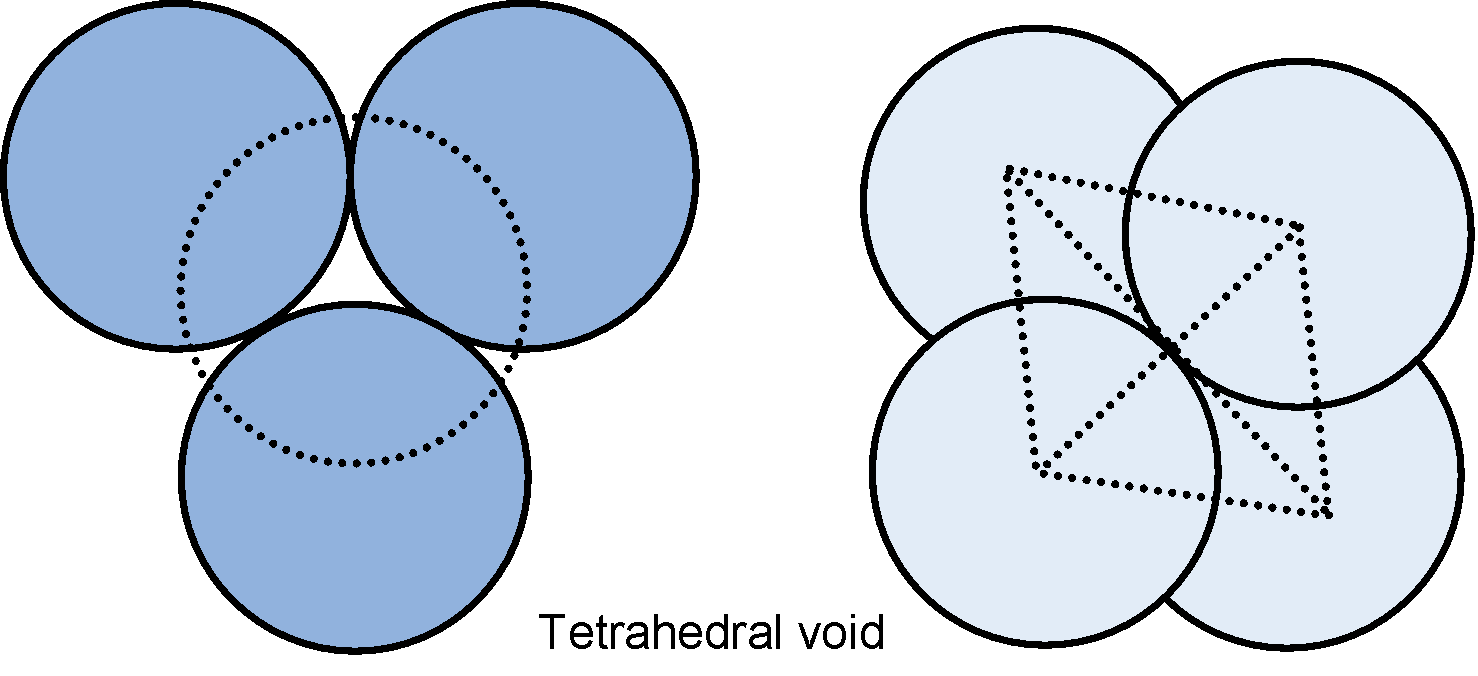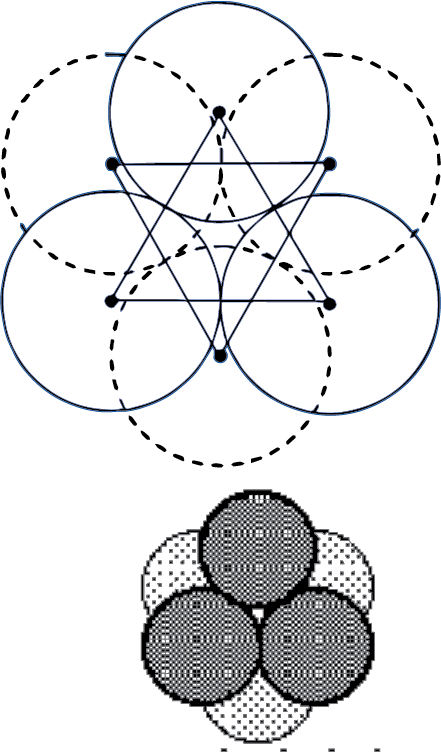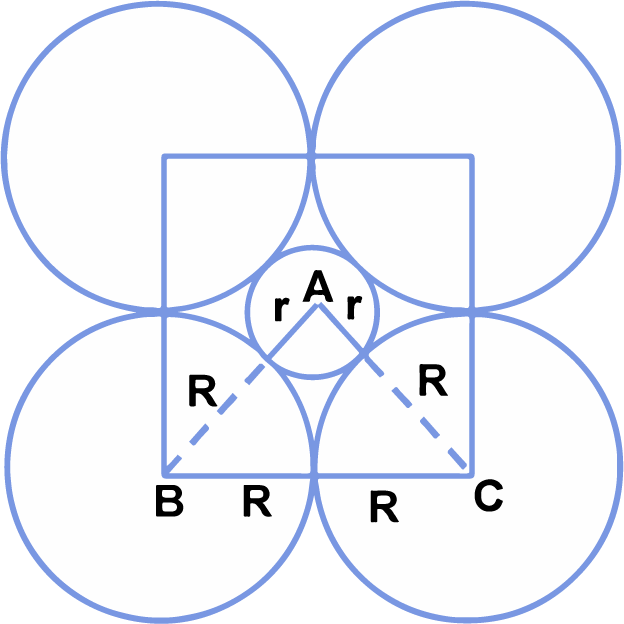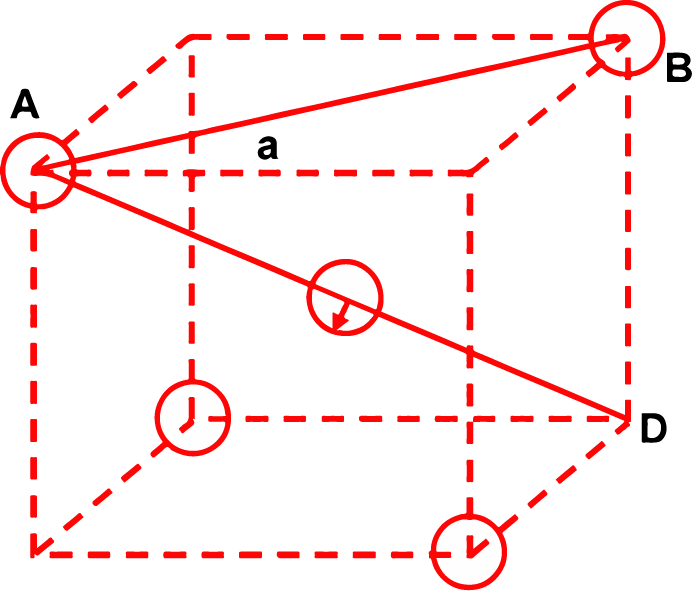# Interstitial Voids

## Interstitial Voids

Vacuum in solid conditions means an empty space in a fully enclosed system between particles. Such empty spaces are known as holes, spaces or interstitial voids. In hcp as well as ccp only 74% of the available space is occupied by spheres. The remaining space is vacant and constitutes interstitial voids or interstices or holes. These are of two types

(a) Tetrahedral voids

(b) Octahedral voids

### Tetrahedral voids

In close packing arrangement, each sphere in the second layer rests on the hollow (triangular void) in three touching spheres in the first layer. The centres of theses four spheres are at the corners of a regular tetrahedral. The vacant space between these four touching spheres is called tetrahedral void. In a close packing, the number of tetrahedral void is double the number of spheres, so there are two tetrahedral voids for each sphereRadius of the tetrahedral void relative to the radius of the sphere is 0.225

i.e. rvoid/rsphere = 0.225

In a multi layered close packed structure , there is a tetrahedral hole above and below each atom hence there is twice as many tetrahedral holes as there are in close packed atoms

### Octahedral voids

As already discussed the spheres in the second layer rest on the triangular voids in the first layer. However, one half of the triangular voids in the first layer are occupied by spheres in the second layer while the other half remains unoccupied. The triangular voids ‘b’ in the first layer are overlapped by the triangular voids in the second layer. The interstitial void, formed by combination of two triangular voids of the first and second layer is called octahedral void because this is enclosed between six spheres centres of which occupy corners of a regular octahedronOctahedral void

In close packing, the number of octahedral voids is equal to the number of spheres. Thus, there is only one octahedral void associated with each sphere. Radius of the octahedral void in relation to the radius of the sphere is 0.414 i.e.  rvoid/rsphere = 0.414

### LOCATING TETRAHEDRAL AND OCTAHEDRAL VOIDS

The close packed structures have both octahedral and tetrahedral voids. In a ccp structure, there is 1 octahedral void in the centre of the body and 12 octahedral void on the edges. Each one of which is common to four other unit cells. Thus, in cubic close packed structure.

Octahedral voids in the centre of the cube =1

Effective number of octahedral voids located at the 12 edge of = 12 x 1/4 = 3

∴ Total number of octahedral voids = 4

In ccp structure, there are 8 tetrahedral voids. In close packed structure, there are eight spheres in the corners of the unit cell and each sphere is in contact with three groups giving rise to eight tetrahedral voidsCircles labelled T represent the centers of the tetrahedral interstices in the ccp arrangement of anions. The unit cell "owns" 8 tetrahedral sites. Circles labelled O represent centers of the octahedral interstices in the ccp arrangement of anions (fcc unit cell). The cell "owns" 4 octahedral sites.

### SIZES OF TETRAHEDRAL AND OCTAHEDRAL VOIDS

(i) Derivation of the relationship between the radius (r) of the octahedral void and the radius (R) of the atoms in close packing.

 A sphere into the octahedral void is shown in the diagram. A sphere above and a sphere below this small sphere have not been shown in the figure. ABC is a right angled triangle. The centre of void is A. Applying Pythagoras theorem.⇒ (√2) = (R + r)2

⇒ (√2R) = (R + r)

⇒ r = √2R - R = (1.414 - 1)R

r = 0.414 R

(ii) Derivation of the relationship between radius (r) of the tetrahedral void and the radius (R) of the atoms in close packing:

To simplify calculations, a tetrahedral void may be represented in a cube as shown in the figure. In which there spheres form the triangular base, the fourth lies at the top and the sphere occupies the tetrahedral void.

 Let the length of the side of the cube = a From right angled triangle ABC, face diagonalAs spheres A and B are actually touching each other, face diagonal AB = 2R ∴ 2R = √2a or R = 1/√2 a ...(i) Again from the right angled triangle ABDBut as small sphere (void) touches other spheres, evidently body diagonal AD = 2(R + r).

∴ 2(R + r) = √3a

⇒ R + r = √3/2a ....(ii)

Dividing equation (ii) by equation (i)⇒ r = 0.225 R

### RADIUS RATIO IN 1:1 OR AB TYPE STRUCTURE

 Radius ratio r+/r− Structural Arrangement Coordination number Example 0.225 – 0.414 Tetrahedral 4 CuCl, CuBr, CuI, BaS, HgS 0.414 – 0.732 Octahedron 6 MgO, NaBr, CaS, MnO, KBr, CaO 0.732 – 1 Cubic 8 CsI, CsBr, TlBr, NH4Br

Q1. What is interstitial voids and  their types?

Ans. There are two types of interstitial voids in a 3D structure: Tetrahedral voids: In cubic close packed structure, spheres of the second layer lie above the triangular voids of the first layer. The three spheres of the first layer was touched by each spheres.

Q2. Is interstitial site a void?

Ans. Interstitial site means the position between the regular position in the list of atoms or ions that other atoms or ions may be occupied. In packaging of spheres, certain hallows or voids are left vacant. These holes or voids in crystals are called interstitial voids or interstitial sites.

Q3. How many interstitial sites are present in BCC?

Ans. The body-centered cubic structure (BCC)  has an atom at all 8 corner positions, and another one at the center of the cube.